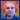Learn
Why Classic?
Start here to get the lowdown on Etherum Classic's reason for being and unique value proposition
Knowledge
Further reading on the foundations that underpin ETC
Guides
Practical tutorials and walkthroughs for interacting with the network in a number of ways
Videos
A collection of videos and podcasts to keep you informed on ETC concepts and happenings
Ethereum Classic Blog

# Ethereum Classic Public And Private Keys: A Little Enlightenment

Christian Seberino

## .css-1r8a28j{-webkit-text-decoration:none!important;text-decoration:none!important;}.css-1r8a28j:hover{-webkit-text-decoration:underline!important;text-decoration:underline!important;}Introduction

Interactions with the Ethereum Classic (ETC) system depend on special numbers referred to as public keys and private keys. Private keys are used to transfer funds, install programs, and, run programs. These numbers must be kept secret at all times. Public keys are used to confirm knowledge of private keys without their disclosure. Public keys also provide a means of identifying accounts.

## Details

Private keys are 32 byte numbers between 1 and

``````115792089237316195423570985008687907852837564279074904382605163141518161494336
``````

inclusive. People randomly select them. Some may be concerned that two people might unintentionally select the same private key. The odds of that happening are vanishingly small. In fact, the number of possible private keys is approximately equal to the number of atoms in the entire universe!

Public keys are 64 byte numbers derived from private keys using an odd type of arithmetic with respect to pairs of numbers. Here is a Python script that calculates public keys from private keys:

``````#!/usr/bin/env python3

"""
Calculates ETC public keys from ETC private keys.

Usage: etc_pub_key <private key>
"""

import random
import sys

A           = 0
N           = 0xfffffffffffffffffffffffffffffffebaaedce6af48a03bbfd25e8cd0364141
P           = 0xfffffffffffffffffffffffffffffffffffffffffffffffffffffffefffffc2f
GX          = 0x79be667ef9dcbbac55a06295ce870b07029bfcdb2dce28d959f2815b16f81798
NUM_FORMAT  = "{{:0{}x}}".format(len(hex(P)[2:]))

def inverse(number):
"""
Inverts a number.
"""

inverse = 1
power   = number
for e in bin(P - 2)[2:][::-1]:
if int(e):
inverse = (inverse * power) % P
power = (power ** 2) % P

return inverse

"""
"""

if   pair_1 == "identity":
sum_ = pair_2
elif pair_2 == "identity":
sum_ = pair_1
else:
if pair_1 == pair_2:
numer   = 3 * pair_1 ** 2 + A
lambda_ = (numer * inverse(2 * pair_1)) % P
else:
numer   = pair_2 - pair_1
denom   = pair_2 - pair_1
lambda_ = (numer * inverse(denom)) % P
x    = (lambda_ ** 2 - pair_1 - pair_2)  % P
y    = (lambda_ * (pair_1 - x) - pair_1) % P
sum_ = (x, y)

return sum_

def multiply(number, pair):
"""
Multiplies a pair by a number.
"""

product = "identity"
power   = pair[:]
for e in bin(number)[2:][::-1]:
if int(e):

return product

def convert(pair):
"""
Converts pairs to numbers by concatenating the elements.
"""

return int("".join([NUM_FORMAT.format(e) for e in pair]), HEXADECIMAL)

print(convert(multiply(int(sys.argv), (GX, GY))))
``````

The reason for this convoluted process is so that private keys cannot be derived from public keys. If you want to learn more, investigate elliptic curve cryptography. The reason for this name is that historically it followed from calculations of the arc lengths of ellipses.

## Miscellany

The first 20 bytes of Keccak 256 hashes of public keys are referred to as account addresses. These are most often used to identify accounts rather than public keys. Interestingly, public keys cannot be determined solely from addresses.

Here is a Python script that calculates addresses from public keys. It requires the PySHA3 package. Addresses are typically expressed in hexadecimal notation and that convention is followed in this script:

``````#!/usr/bin/env python3

"""
Calculates ETC addresses from ETC public keys.

"""

import sha3
import binascii
import sys

N_PUB_KEY_BYTES = 64

public_key = (int(sys.argv)).to_bytes(N_PUB_KEY_BYTES, byteorder = "big")
``````

### Signatures

Private keys provide a way to effectively sign transactions. For this, ETC uses the Elliptic Curve Digital Signature Algorithm (ECDSA). The basic idea is to create sets of numbers, corresponding to transactions, that can only be generated by the sending account private keys. This is analogous to handwritten signatures that can only be generated by the corresponding hands.

### Programs

Programs, or smart contracts, do not have their own public and private keys. Therefore, programs cannot digitally sign transactions. Furthermore, program account addresses must be selected by some other means.

## Example

Here is a slightly edited session, on a Linux computer, that calculates a public key and address with regards to a random private key. It uses the aforementioned scripts saved in files called etc_pub_key and etc_address respectively:

``````% PRIVATE_KEY="92788259381212812445638172234843282167646237087212249687358593145563035518424"

% PUBLIC_KEY=`etc_pub_key \$PRIVATE_KEY`

% echo \$PRIVATE_KEY
92788259381212812445638172234843282167646237087212249687358593145563035518424

% echo \$PUBLIC_KEY
9808854183897174607002157792089896992612613490844656534725423301978228163634425857099752732031947328803605451685330420628756154476771607661633738743568351

89b44e4d3c81ede05d0f5de8d1a68f754d73d997
``````

## Conclusion

Public and private keys are widely used in ETC and in other technologies. Therefore, I hope this has been enlightening.

## Feedback

You can contact me by clicking any of these icons:

## Acknowledgements

I would like to thank IOHK (Input Output Hong Kong) for funding this effort.cseberino
The ETC community is active on Discord
Github
Discord
Reddit
Made with `<3` for the Original Ethereum Vision